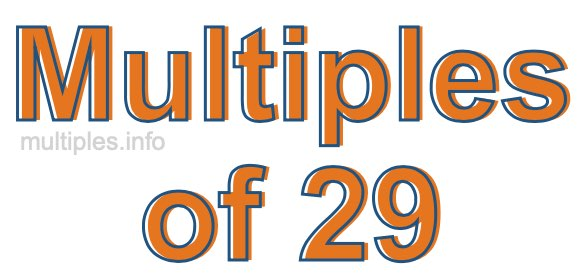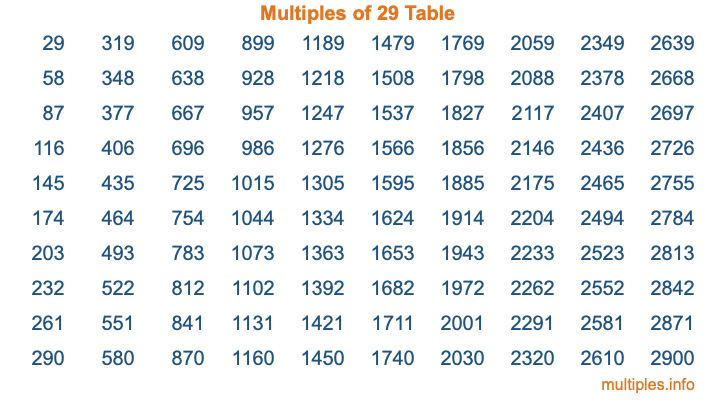Multiples of 29Welcome to the Multiples of 29 page. Here we will first teach you everything you will ever need to know about the multiples of 29, and then give you a study guide summary of everything we taught you to make sure you remember it all. Use this page to look up facts and learn information about the multiples of 29. This page will make you a multiples of twenty-nine expert!

Definition of Multiples of 29
Multiples of 29 are all the numbers that when divided by 29 equal an integer. Each of the multiples of 29 are called a multiple. A multiple of 29 is created by multiplying 29 by an integer.

Therefore, to create a list of multiples of 29, you start with 1 multiplied by 29, then 2 multiplied by 29, then 3 multiplied by 29, and so on for as long as you want. Thus, the list of the first five multiples of 29 is 29, 58, 87, 116, and 145. To see a larger list of multiples of 29, see the printable image of Multiples of 29 further down on this page. We also have a category where you can choose any nth multiple of 29.

Multiples of 29 Checker
The Multiples of 29 Checker below checks to see if any number of your choice is a multiple of 29. In other words, it checks to see if there is any number (integer) that when multiplied by 29 will equal your number. To do that, we divide your number by 29. If the the quotient is an integer, then your number is a multiple of 29.

Is  a multiple of 29?

Least Common Multiple of 29 and ...
A Least Common Multiple (LCM) is the lowest multiple that two or more numbers have in common. This is also called the smallest common multiple or lowest common multiple and is useful to know when you are adding our subtracting fractions. Enter one or more numbers below (29 is already entered) to find the LCM.

Check out our LCM Calculator if you need more details about the Least Common Multiple or if you need the LCM for different numbers for adding and subtraction fractions.

nth Multiple of 29
As we stated above, 29 is the first multiple of 29, 58 is the second multiple of 29, 87 is the third multiple of 29, and so on. Enter a number below to find the nth multiple of 29.

th multiple of 29

Multiples of 29 vs Factors of 29
29 is a multiple of 29 and a factor of 29, but that is where the similarities end. All postive multiples of 29 are 29 or greater than 29. All positive factors of 29 are 29 or less than 29.

Below is the beginning list of multiples of 29 and the factors of 29 so you can compare:

Multiples of 29: 29, 58, 87, 116, 145, etc.

Factors of 29: 1, 29

As you can see, the multiples of 29 are all the numbers that you can divide by 29 to get a whole number. The factors of 29, on the other hand, are all the whole numbers that you can multiply by another whole number to get 29.

It's also interesting to note that if a number (x) is a factor of 29, then 29 will also be a multiple of that number (x).

Multiples of 29 vs Divisors of 29
The divisors of 29 are all the integers that 29 can be divided by evenly. Below is a list of the divisors of 29.

Divisors of 29: 1, 29

The interesting thing to note here is that if you take any multiple of 29 and divide it by a divisor of 29, you will see that the quotient is an integer.

Multiples of 29 Table
Below is an image of the first 100 multiples of 29 in a table. The table is in chronological order, column by column. The first column has the first ten multiples of 29, the second column has the next ten multiples of 29, and so on.The Multiples of 29 Table is also referred to as the 29 Times Table or Times Table of 29. You are welcome to print out our table for your studies.

Negative Multiples of 29
Although not often discussed or needed in math, it is worth mentioning that you can make a list of negative multiples of 29 by multiplying 29 by -1, then by -2, then by -3, and so on, to get the following list of negative multiples of 29:

-29, -58, -87, -116, -145, etc.

Multiples of 29 Summary
Below is a summary of important Multiples of 29 facts that we have discussed on this page. To retain the knowledge on this page, we recommend that you read through the summary and explain to yourself or a study partner why they hold true.

There are an infinite number of multiples of 29.

A multiple of 29 divided by 29 will equal a whole number.

29 divided by a factor of 29 equals a divisor of 29.

The nth multiple of 29 is n times 29.

The largest factor of 29 is equal to the first positive multiple of 29.

29 is a multiple of every factor of 29.

29 is a multiple of 29.

A multiple of 29 divided by a divisor of 29 equals an integer.

29 divided by a divisor of 29 equals a factor of 29.

Any integer times 29 will equal a multiple of 29.

Multiples of a Number
Here you can get the multiples of another number, all with the same attention to detail as we did for multiples of 29 on this page.

Multiples of
Multiples of 30
Did you find our page about multiples of twenty-nine educational? Do you want more knowledge? Check out the multiples of the next number on our list!